# Volume Of A Cube

Here we will learn about the volume of a cube, including how to calculate the volume of a cube and how to find missing lengths of a cube given its volume.

There are also volume of a cube worksheets based on Edexcel, AQA and OCR exam questions, along with further guidance on where to go next if you’re still stuck.

## What is the volume of a cube?

The volume of a cube is the amount of space there is within a cube.

A cube is a three-dimensional shape with 6 square faces.

To find the volume of a cube, with side length a, we can use the volume of a cube formula,

\text {Volume }=a^{3}.

Volume is measured in cubic units for example, mm^{3}, \ cm^{3} or m^{3}.

For example,

The volume of this cube is

\begin{aligned} \text{Volume} &=a^{3} \\\\ \text{Volume} &=8^{3} \\\\ \text{Volume} &=512 \mathrm{~cm}^{3} \end{aligned}

### What is the volume of a cube?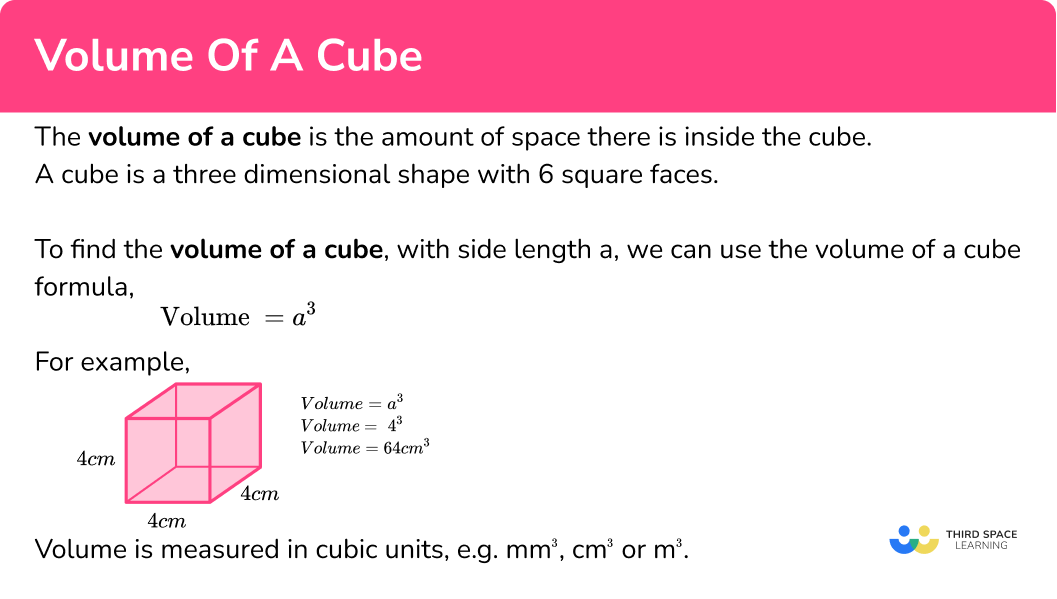## How to calculate the volume of a cube

In order to calculate the volume of a cube:

1. Write down the formula.

\text{Volume }=a^{3}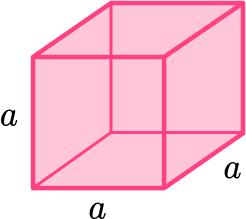2. Substitute the values into the formula.
3. Work out the calculation.
4. Write the answer and include the units.

### Explain how to calculate the volume of a cube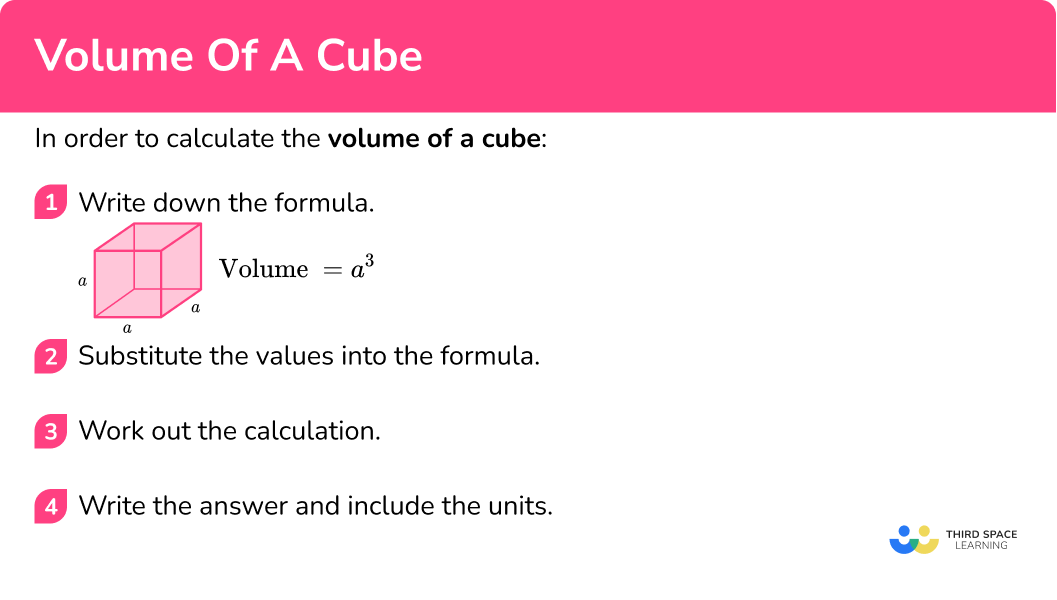## Volume of a cube examples

### Example 1: volume of a cube

Work out the volume of the cube.

1. Write down the formula.

\text{Volume }=a^{3}

2Substitute the values into the formula.

Here the sides of the cube are 6cm.

\text{Volume }=6^{3}

3Work out the calculation.

\begin{aligned} \text{Volume} &=6 \times 6 \times 6\\\\ &=216 \end{aligned}

4Write the answer and include the units.

The measurements are in cm therefore the volume will be in cm^{3}.

\text{Volume }=216 \mathrm{~cm}^{3}

### Example 2: volume of a cube

Work out the volume of this cube.

Write down the formula.

Substitute the values into the formula.

Work out the calculation.

Write the answer and include the units.

### Example 3: volume of a cube – different units

Work out the volume of this cube.

Write down the formula.

Substitute the values into the formula.

Work out the calculation.

Write the answer and include the units.

## How to work out a missing length given the volume

Sometimes we are given the volume of a cube and need to work out the length of an edge.

We can do this by substituting the volume into the formula for the volume of a cube and then solving the equation that is formed. This will give us the length of the sides of a cube.

1. Write down the formula.
\text{Volume }=a^{3}
2. Substitute the values that you do know into the formula.
3. Solve the equation.
4. Write the answer and include the units.

## Finding the length given the volume examples

### Example 4: find the length of a cube given the volume

The volume of the cube is 64 \ cm^{3}. Work out the length of the cube.

Write down the formula.

Substitute the values that you do know into the formula.

Solve the equation.

Write the answer and include the units.

### Example 5: find the length of a cube given the volume

The volume of the cube is 1000 \ m^{3}. Work out the length of each side, x.

Write down the formula.

Substitute the values that you do know into the formula.

Solve the equation.

Write the answer and include the units.

### Common misconceptions

• Missing/incorrect units

Volume is measured in units cubed (e.g. mm^{3}, \ cm^{3}, \ m^3 etc).

• Calculating with different units

You need to make sure all measurements are in the same units before calculating volume.

For example, you can’t have some in cm and some in m.

• Dividing by three rather than cube rooting

If you are given the volume of a cube and you need to find the side length, remember the inverse of cube is cube root, not divide by 3.

For example, if the volume of a cube is 27 \ cm^{3}, the side length is \sqrt{27}=3 \ \mathrm{cm} (not 27 \div 3 ).

Surface area of a cuboid is part of our series of lessons to support revision on cuboid. You may find it helpful to start with the main cuboid lesson for a summary of what to expect, or use the step by step guides below for further detail on individual topics. Other lessons in this series include:

### Practice volume of a cube questions

1. Work out the volume of the cube.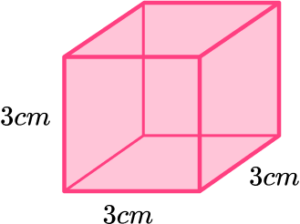9 \ \mathrm{cm}^{3}27 \ \mathrm{cm}^{3}54 \ \mathrm{cm}^{3}36 \ \mathrm{cm}^{3}\begin{aligned} \text{Volume } &=a^{3} \\\\ \text{Volume } &=3^{3} \\\\ &=3 \times 3 \times 3 \\\\ &=27 \mathrm{~cm}^{3} \end{aligned}

2. Work out the volume of the cube.1.25 \ \mathrm{m}^{3}150 \ \mathrm{m}^{3}125 \ \mathrm{m}^{3}0.125 \ \mathrm{m}^{3}\begin{aligned} \text{Volume }&=a^{3}\\\\ \text{Volume }&=0.5^{3}\\\\ &=0.5 \times 0.5 \times 0.5\\\\ &=0.125 \mathrm{~m}^{3} \end{aligned}

3. Work out the volume of this cube.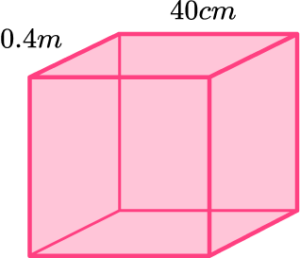64000 \ \mathrm{cm}^{3}96 \ \mathrm{cm}^{3}64 \ \mathrm{cm}^{3}6.4 \ \mathrm{m}^{3}Notice here that one of the units is in cm whilst the other is in m . We need all the units to be the same to calculate the volume.

This is a cube, we know all the edges are the same length, so we can easily change m to cm, \ 0.4 \ m = 40 \ cm.

\begin{aligned} \text{Volume }&=a^{3}\\\\ \text{Volume }&=40^{3}\\\\ &=40 \times 40 \times 40\\\\ &=64000 \end{aligned}

The measurements are in cm therefore the volume will be in cm^{3}.

\text{Volume }=64000 \ \mathrm{~cm}^{3}

4. The volume of a cube is 343 \ cm^{3}. Find the length of the side.

7 \ \mathrm{cm}^{3}114.3 \ \mathrm{~cm}7 \ \mathrm{~cm}114.3 \ \mathrm{m}^{3}\text{Volume }=a^{3}

\begin{aligned} a^{3}&=343 \\\\ a&=\sqrt{343} \\\\ a&=7 \mathrm{~cm} \end{aligned}

5. The volume of the cube is 125 \ m^{3}. Work out the length of each side x.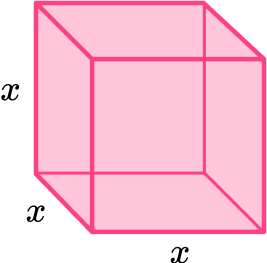5 \ \mathrm{~m}41.6 \ \mathrm{m}^{3}41.6 \ \mathrm{~m}5 \ \mathrm{m}^{3}\text{Volume }=x^{3}

\begin{aligned} x^{3}&=125 \\\\ x&=\sqrt{125} \\\\ x&=5 \mathrm{~m} \end{aligned}

6. The volume of a cube is 64 \ m^{3}. Work out the length of each side. Give your answer in cm.

4 \ \mathrm{~m}21.3 \ \mathrm{m}^{3}21.3 \ \mathrm{~m}400 \ \mathrm{~cm}\text{Volume }=a^{3}

\begin{aligned} a^{3}&=64 \\\\ a&=\sqrt{64} \\\\ a&=4 \mathrm{~m} \end{aligned}

4m = 400 \ cm

a=400 \mathrm{~cm}

### Volume of a cube GCSE questions

1. Here is a cube.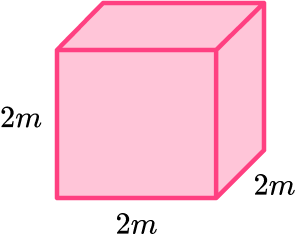Work out the volume of the cube.

(2 marks)

\text{Volume}=2 \times 2 \times 2

(1)

\text{Volume}=8 \ \mathrm{m}^{3}

(1)

2. (a) This sculpture is formed by placing one cube on top of another. Work out the total volume of the sculpture.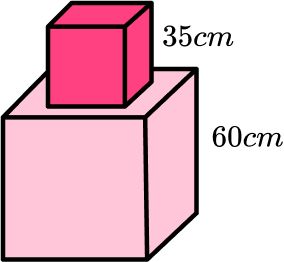(b) The density of the material used to make the sculpture is 2.7 \ g/cm^{3}. Work out the total mass of the sculpture. Give your answer in kg, to the nearest kg.

You may use \text{density }= \frac{\text{mass}}{\text{volume}} .

(5 marks)

(a)

Volume of bottom cube = 60 \times 60 \times 60=216000 \ cm^3

(1)

Volume of top cube = 35 \times 35 \times 35=42875 \ cm^3

(1)

Total volume = 216000+42875=258875 \ cm^3

(1)

(b)

2.7=\frac{m}{258875}

(1)

2.7 \times 258875=698962.5 \ \mathrm{g}

(1)

698962.5 \ g=698.9625 \ kg=699 \ kg (nearest kg )

(1)

3. A cube has a volume of 343 \ m^{3}. Find the length of the cube. Give your answer in cm.

(3 marks)

x^{3}=343 or x=\sqrt{343}

(1)

x= 7 \ m

(1)

x= 700 \ cm

(1)

## Learning checklist

You have now learned how to:

• Understand and apply the formula to calculate the volume of cubes
• Use the properties of faces, surfaces, edges and vertices of cubes and cuboids to solve problems in 3D

## Still stuck?

Prepare your KS4 students for maths GCSEs success with Third Space Learning. Weekly online one to one GCSE maths revision lessons delivered by expert maths tutors.

Find out more about our GCSE maths tuition programme.